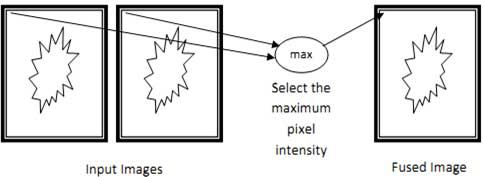## Matlab code for Pixel level Image Fusion using Minimum Method

6,000.00

Matlab code for Pixel level Image fusion using Minimum Method

100 in stock

SKU: Image-Fusion-Minimum-Method Category:

## Matlab code for Pixel level Image fusion using Minimum Method

The minimum selecting method, being yet another trivial image fusion method, is very similar to the Maximum Selection method; except for, here, the selection criteria differs as the pixel with minimum density is picked up. Thus, for every pixel position, the pixel of the fused image will be the pixel of the corresponding position from the input set of images having the least pixel intensity value. Similar to the Maximum Selection method, this method to either completely considers the information from an input image or discards it fully. No averaging or any operation of the like is performed here. The quality of the fusion is specific to the type of image we are dealing with. In certain cases, say, images with dark shades would generate a good fusion image with this method. The stepwise description of the algorithm is discussed below

## Demonstration Video

• Compare the intensity value of the corresponding pixels of the input pair of images.
• Generate the selection matrix based on the comparison performed in 1, assigning value 0 for condition being true and 1 otherwise
• Multiply the corresponding value in the selection matrix with first image matrix
• Multiply the corresponding value in the negated selection matrix with second image matrix.
• Resultant image matrix id calculated by adding the matrices calculated in 3 and 4.The maximum of the pixel intensities at every position (m,n) is selected as the (m,n) pixel of the fused image

```clc;

clearall;

closeall;

M1=double(M1);

M2=double(M2);

mm = M1 < M2;

FusedImage = (mm.*M1) + ((~mm).*M2);

subplot(1,3,1);

imshow(M1,[ ]);

subplot(1,3,2);

imshow(M2,[ ]);

title('Image Fusion using Minimum');

subplot(1,3,3);imshow(FusedImage,[ ]);
```

## Reviews

There are no reviews yet.

This site uses Akismet to reduce spam. Learn how your comment data is processed.